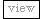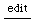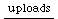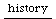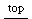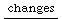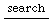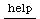## Triggering Sound Events (SC2)

back to Theory

### A) Synthesis of Trigger Signals

the most basic Trigger UGens are
• Impulse (short peak at regular intervals)
• Dust/Dust2 (short peak at random intervals and random levels)

In many UGens a "trigger happens when the signal changes
from non-positive to positive", so basically
any contiuous output can form a trigger.

to get Impulses from any signal, one can also use
• > (larger than)
• < (smaller than)
• HPZ1 / HPZ2 (outputs an impulse depending on the slope of a signal)
```(
//HPZ example:
{       a = LFNoise0.ar(1000);
b = Lag.ar(a, Line.kr(0, 0.0008, 0.01));
c = HPZ2.ar(a);
d = HPZ1.ar(a);
e = HPZ2.ar(b);
f = HPZ1.ar(b);

[a,c*3, d*3, b, e*3, f*3];
}.plot;
)

```

by James McCartney for an interesting way to use HPZ1

To trigger from a GUI, one can use a Plug, gate it from the action method and make a trigger using > 0:

```
(
//Trigger GUI
var p, trig;

w = GUIWindow.new("", Rect.newBy(-13, 342, 128, 100));
trig = ButtonView.new( w, Rect.newBy(33, 35, 55, 34), "trig", 1, 0, 1, 0, 'linear');

trig.action = { p.gate(1, 0.1) };

{
var sig;
p = Plug.kr(0);
sig = p > 0;
TSpawn.ar({
PSinGrain.ar(1000+300.rand, 0.01, 0.1)
}, 1, nil, sig);
}.play;
)

//note that the plug has to be declared globally ( var p ),
//but assigned within a synth (p = Plug.kr(0))
```

### B) Processing Trigger Signals

• ImpulseSequencer uses a function or a stream to assign an amplitude to each trigger. The use of Streams opens up a wide range of possibilities
• Multiply a Control Rate UGen with the trigger signal
Triggers can be processed by the various Trigger UGens, to be found at http://www.audiosynth.com/schtmldocs/Help/Unit_Generators/Triggers/
• To increase the number of Trigger Impulses, one can use Delays like MultiTap.
• To reduce the number of Trigger Impulses, one can use a PulseDivider
or something like the following:
```(
var route;
route = Routine.new({ loop({
16.do({ arg i;
(i * 4 + 8).do({ arg p;
if(p.isPrime, { 1.yield }, { 0.yield })
});
16.do({ arg i; (1-(i/16)).yield })
})
})     });
{ var trig, freq;
freq = MouseX.kr(2, 30);
trig = ImpulseSequencer.ar(route, Impulse.ar(freq));
Decay2.ar(trig, 0.02/freq, 0.1/freq, FSinOsc.ar(1000, 0.1));
}.play;
)
)
```

### C) Triggering Sound Events

#### Triggering sounds:

• use an instance of TSpawn (like above), e.g.TrigXFade
• lengthen the trigger impulse and multiply it with a continuous sound : Trig, Decay / Decay2 ( decayexample ), EnvGen
• also see: Processing Trigger Signals

example:
```(
{
var trig, output;
trig = Dust.kr(8);
output = Trig.kr(trig, MouseX.kr(0, 0.7));
output = output * SinOsc.ar(400, 0, 0.1)
}.play;
)
```

Note that Control rate and Audio rate makes a difference sometimes:
{K2A.ar(Impulse.kr(200))}.plot; //K2A is used here to translate control rate to audio rate
{ Impulse.ar(200, 0.2) }.plot;

#### Triggering other actions:

A sequencer UGen can be used to evaluate any function when triggered.
Note that the first evaluation happens on creation of Sequencer, not on the first trigger signal.

In order to avoid this, use the counter argument:
```
Sequencer.kr({ arg i; if(i != 0, { function }); 0.0}, trig)
```

a Sequencer Stream must return discrete values, so function must return an integer or a float (0.0)

please feel free to contribute
how to contribute (redirected) to this swiki

authors so far:
jrh
...